### Spectral estimation of noise

In the first part on spectral estimation, we learned different techniques to estimate the Fourier transform of a given signal.  We saw that the main issues were related to the fact that from the original continuous signal, we could only obtain and treat digitally a finite number of sample values:

1. One could only obtain a sample every Ts seconds because any electronics takes finite time to do so and transmit the data.
2. One could only obtain a sample for a period of time T because we have to start at a certain moment, and we have to stop at a certain moment in order to be able to even start to treat the data

These limitations of the data we had concerning the a priori infinite duration, and continuous large bandwidth signal, resulted in two effects:

1. Aliasing (related to the finite sampling frequency)
2. Leakage (related to the finite period of sampling)

In this part, we will finally come to what we set out in the beginning: estimate the spectral density of noise.  That is, estimate the spectral density of an ensemble of signals.  We see that there is now a supplementary difficulty: we were already not able to find the right Fourier Transform of a single, given, deterministic signal because of aliasing and leakage due to the finite amount of samples that we could gather.  Now, we will not even have all the signals of the ensemble to our disposition to sample from but only one single realisation !

Of course, we will make two assumptions, without which it would be impossible to even start to try to estimate any spectral density of the ensemble.  These assumptions are:

1. Stationarity
2. Ergodicy

Stationarity means that there is no absolute time dependence.  This means that the statistical properties remain conserved over time.  The spectral density doesn't even make sense without stationarity.

Ergodicity means that averages over a single realisation in time converge to ensemble averages.  Without this hypothesis, the only single realisation of the ensemble that we have at our disposition would not convey the information (ensemble statistical properties) we're after.  Fundamental physical noise usually has these properties of stationarity and ergodicity.  Pick up noise doesn't in most cases.

Under the assumptions of stationarity and ergodicity, one can hope to get an estimator for the spectral density of the ensemble based upon sufficient samples of a single realisation of that ensemble.  However, there are some naive caveats.

If one has a recorded, sampled piece of noise signal, then naively one could think that one obtains the spectral density of it by calculating its DFT.   One is then usually surprised to find that one has a very noisy, essentially unusable "spectrum".  This shouldn't surprise us:  if one takes the DFT of a realisation of noise, one has a single realisation of the ensemble of Fourier transforms of that noise.   This ensemble is just as noisy as the original noise !

The noise spectral density, however, will be the (rms) average of the amplitude of these Fourier transform realisations of that ensemble.  One can indeed consider the ensemble of Fourier transforms of the original ensemble and look at its statistical description.   It will then turn out that:

1. The phases are uniformly distributed between 0 and 2 π
2. The amplitudes squared are distributed with an average given by the spectral power density

These properties are exact for the ensemble of the abstract, mathematical Fourier transforms of the full, infinite-duration noise realisations of the original ensemble, on the condition that the original ensemble is stationary.

### The periodogram

The idea is in fact already outlined above: we would like to take the average of the (squared) amplitude of the Fourier transform over the ensemble of signals, as this is exactly what the spectral density of that ensemble is. However, we will have to make several approximations to make this practical:

• We will have to limit ourselves to a DFT for a given representative of the signal ensemble.

• We will have to limit ourselves to one single representative and use ergodicity to take the average over the ensemble, and we will have to make a finite averaging estimator.

In other words, considering that we have only one single representative of the signal, we will have to:

• take M finite chunks of that single representative

• sample those chunks (obtaining 2 N samples for each of them)

• calculate the DFT of each of these M sequences of 2 N samples

• calculate the average over the M different amplitudes squared for each of the N frequencies.

This last average is considered our estimate of the spectral power density at the N frequencies corresponding to the DFT we took.  The above method is called Bartlett's periodogram method, and the standard non-parametric spectral estimation method.

However, the different approximations that are taken, will have the potential to introduce systematic problems in the obtained estimation, and we will discuss these now. A very sensitive indicator will be the phase relationship between different frequency components. Indeed, as we argued earlier, true stationary noise has, for each frequency component in the Fourier transform, a phase which is uniformly distributed between 0 and 2 pi (or between minus pi and pi). But also, all these phases of different frequency components are uncorrelated. The reason is that if there were a phase relationship between these phases, this would indicate statistically preferred moments in time over others, which is impossible under the hypothesis of stationarity. As linear filters only introduce a phase shift for each frequency component, the distribution of the phases remains uniform between 0 and 2 pi and the phases of different frequency components remain uncorrelated, even if a linear filter is applied to stationary noise.

We will see that the ensemble of estimated DFT of such a filtered signal doesn't agree with this fundamental property, illustrating effects of these approximations.

### Pseudo randomly generated uniform white noise samples.

We first look at the simplest case of simulated white noise samples. No matter how one samples those, we will simply end up with statistically independent random numbers, distributed according to the amplitude distribution of the noise, in this case, uniform between -0.5 and 0.5. We would expect the DFT of a sequence of such numbers to be just as independent and random (the DFT being a unitary transformation).

We have generated 10 000 sequences (M) of 512 (2 N) random numbers. We then take the DFT of each of these sequences, giving us essentially 256 frequency points with an amplitude and a phase for each frequency. The 10 000 sequences are a statistical sampling of the ensemble of DFT from which we are going to calculate the averages, and their study in more detail will reveal some expected and unexpected effects.

The distribution of the phase of the third and the 80th frequency component (which are expected to be uniformly distributed) is nicely uniform as it should be. If it isn't, there is a problem with the statistical independence of the random number generator. Note that the correlation between the phases between different frequency components is perfectly uncorrelated, as expected.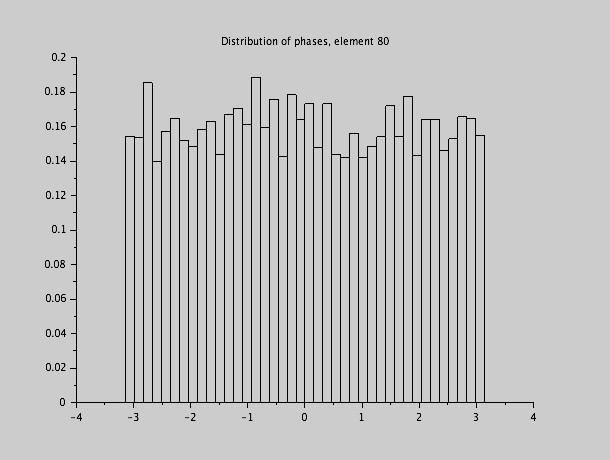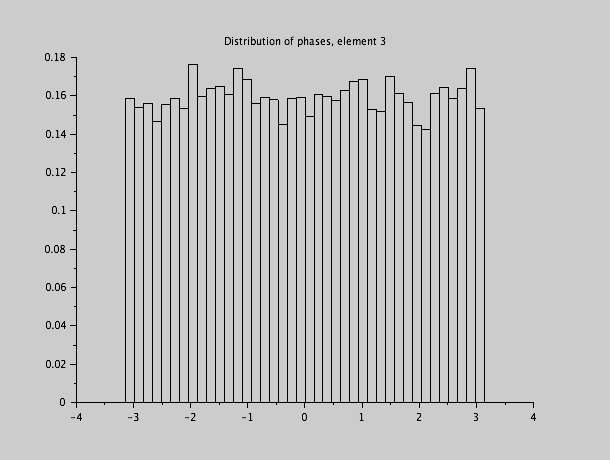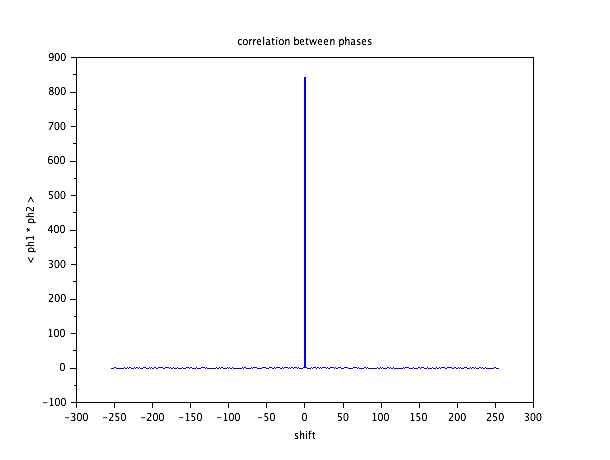As we have a r.m.s. value of the individual samples equal to 1/ sqrt(12) (a uniform distribution with width equal to 1), and we have 512 samples, we expect an average frequency amplitude of sqrt(512) / sqrt(12) = 6.53

So we expect an amplitude distribution more or less centered around 6.53.

The histogram of the amplitude distribution of the 80th and the 3rd frequency component is shown.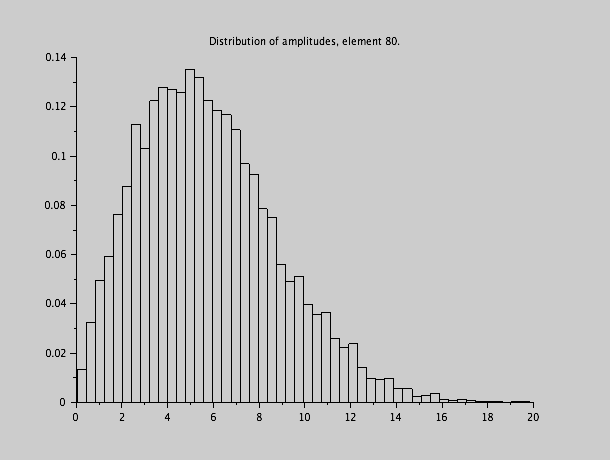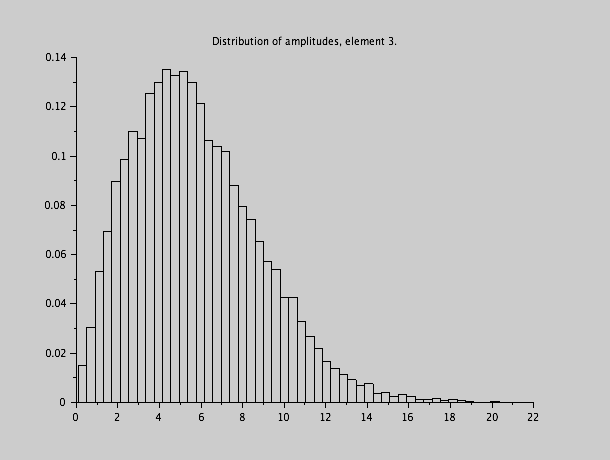We also expect the individual deviations of the actual amplitude from the average to be uncorrelated from frequency component to frequency component. This is indeed the case.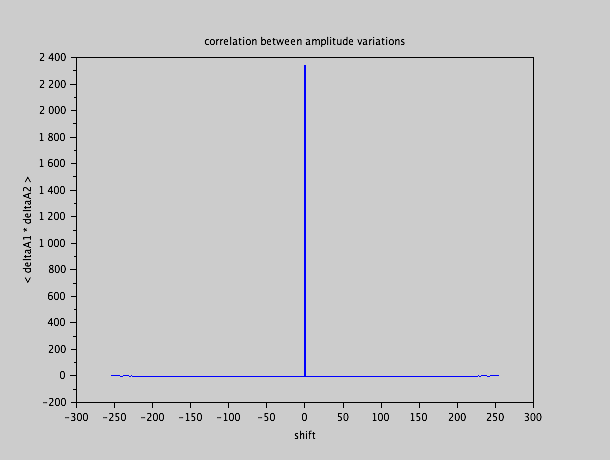Finally, the average of the distribution of amplitudes, phases, the real part and the imaginary part of the frequency component is shown. For white noise, we expect this to be constant. It is indeed the case.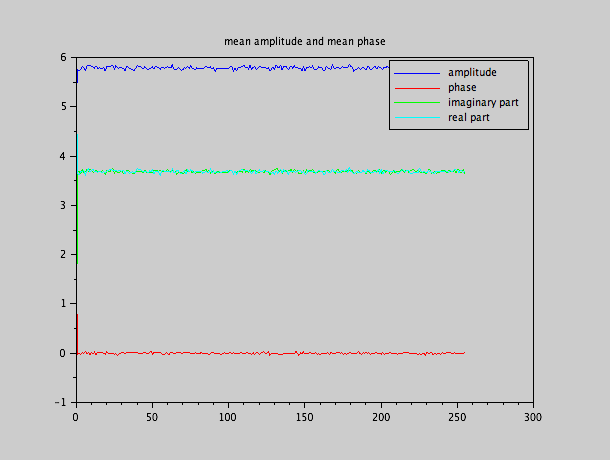Next, we pass this white noise through a linear filter, in order for us to study a non-trivial spectral power density. We use an elliptic band pass filter of order 4 to this end. Of course, we have to take care to send first some noise signal to the filter before starting to sample, as we want to avoid taking the “starting phase” of the filter, which would ruin the time-invariance.

As indicated before, the phases should still remain uniformly distributed and uncorrelated between different frequency components. It turns out that this is not the case.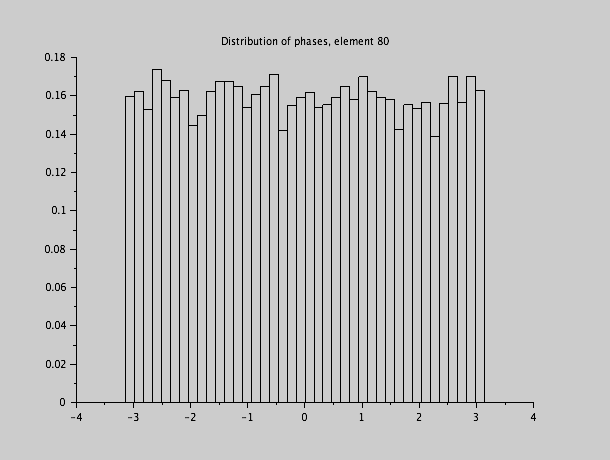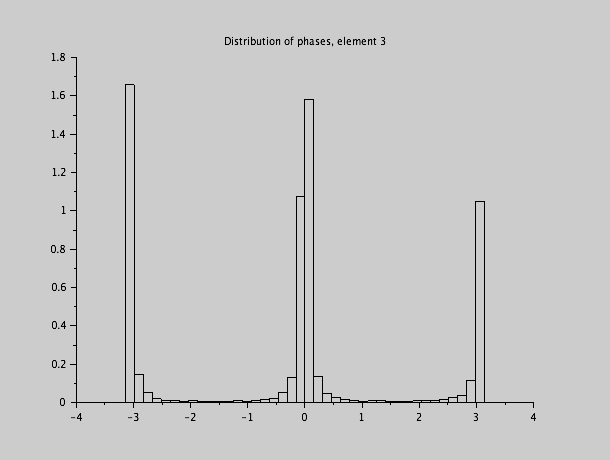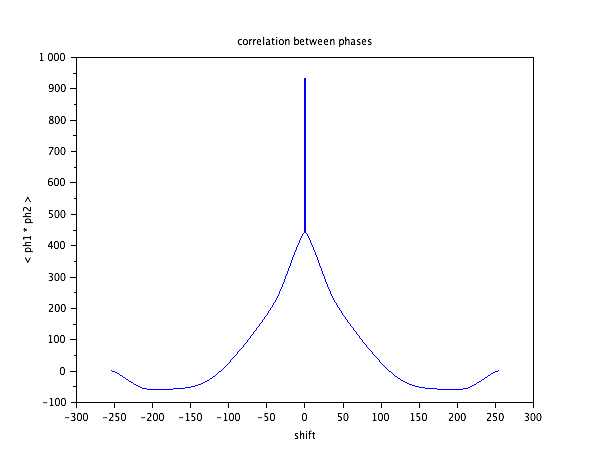It is interesting to note that the frequency component illustrated here, which has the wrong phase distribution (component 3) is in the stop band of the filter, while the frequency component that still has the right distribution (component 80) is in the pass band, as can be seen in the averages: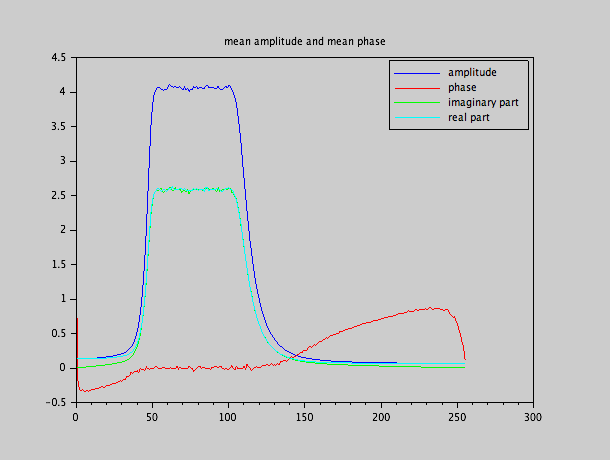Note also the deviation of the real part of the frequency component in the part of the stop band near 0 frequency: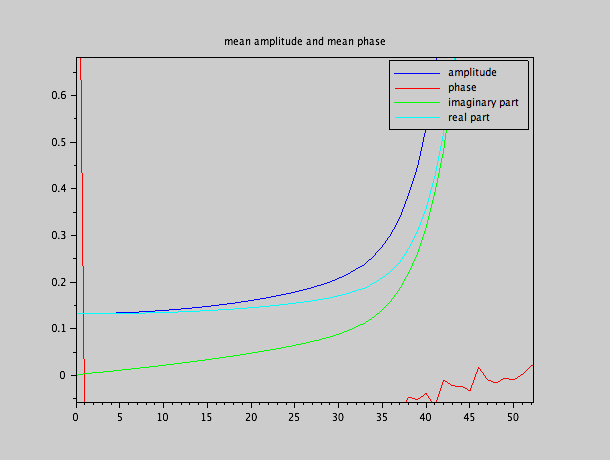We see that the real part of the spectral components is "too high", while the imaginary component doesn't seem to be influenced.   This lack of correct value of this real part is what ruins the uniform phase distribution too.

What we obtain here, is in fact leakage.  By definition, noise is not periodic, and has as such a continuous frequency spectrum.  As illustrated, taking the DFT of a non-periodic signal will give rise to leakage.    However, how is it possible that there is a distinction between the real and the imaginary components ?  That would indicate that our signal is not time-invariant.  In fact, leakage introduces a time-dependent effect correlated to the absolute time position of the window in which the samples are taken.

We can show this by artificially making the noise "periodic".  As such, we destroy of course the statistical time invariance property of the noise, and this would also mean that we cannot use our technique to measure the power spectral density of a given signal.  The filter here was only used to produce a noise sequence with non-trivial spectral density, but in reality we don't have access to the pre-filter sequence.  Nevertheless, if we repeat several times the same white noise sequence before filtering it, the input signal of the filter has become of course periodic, and its output will be, too.

Looking at the phase distributions and correlation in that case: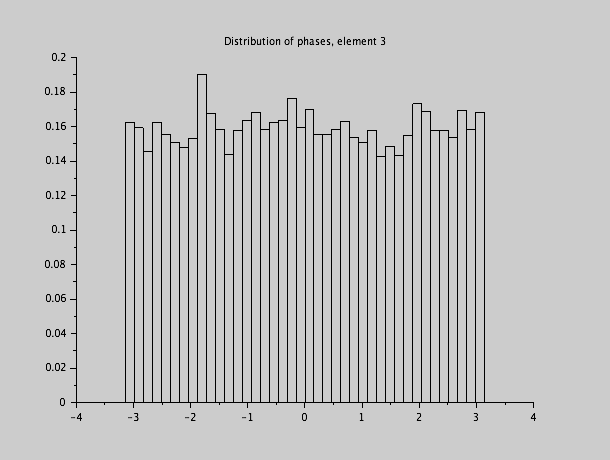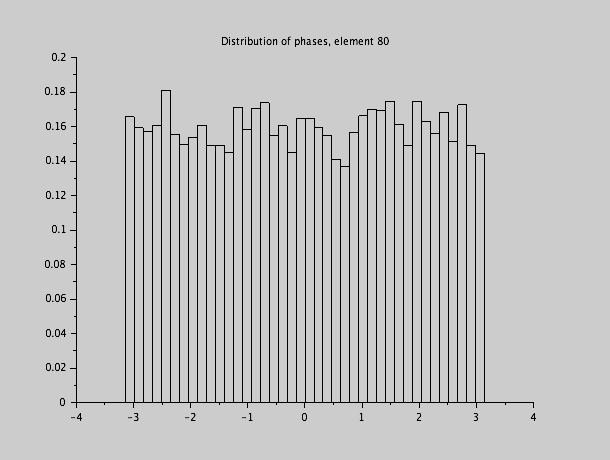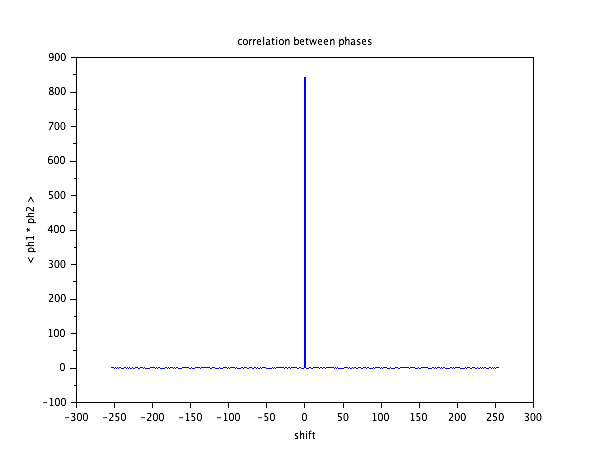The averages per frequency component are now also as expected: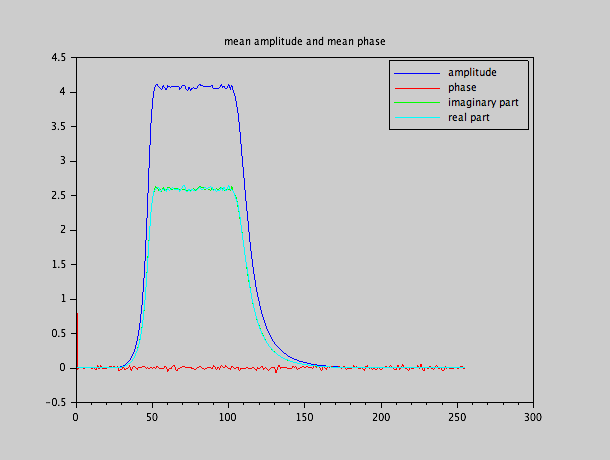As we see, the fact of fabricating artificially periodic noise has solved all the issues.  But as pointed out, this is not something that can be done in practice: we cannot turn the noise under study into a genuine periodic signal of course !  We could only do this in this example because we were simulating the noise ourselves, and hence had our hands on the source of the noise.

Nevertheless, it proves sufficiently that the phase problem, and even the amplitude problem, was due to the (unavoidable) leakage of the continuous frequency properties of genuine noise.  As we have seen, the actual solution to leakage is to use a good windowing function.  We have been using a rectangular window in our case, and it is in reality much better to use another windowing function that limits the "far away leakage".   Of course, introducing a window function will not solve entirely the problems of leakage.  But we will show here that for the case at hand, using a Blackman window solves most of the issues.

Indeed, going back to the "genuine noise" (that is, not repeating the input sequence to the filter any more), but applying a Blackmann window, we obtain the following phase results: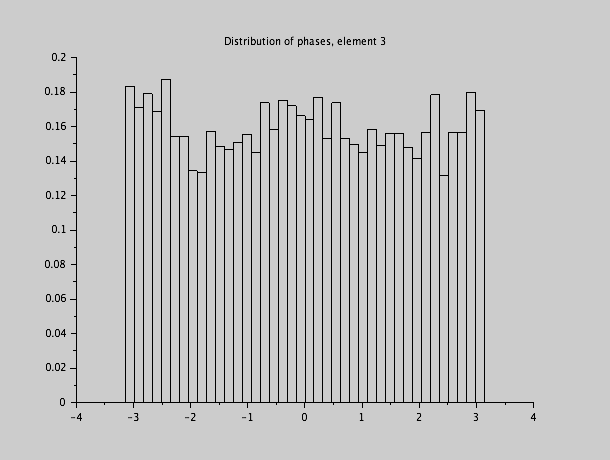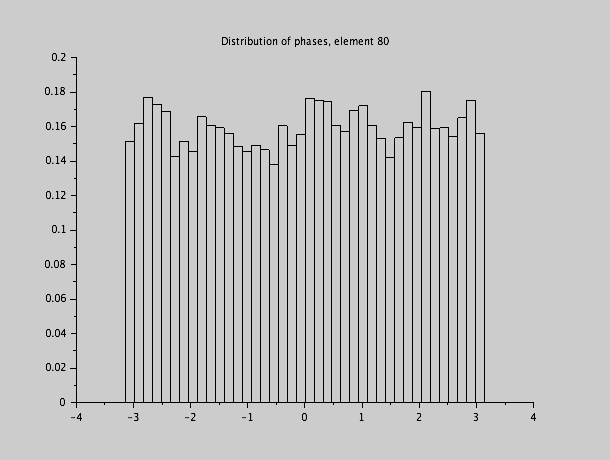Their correlations are now: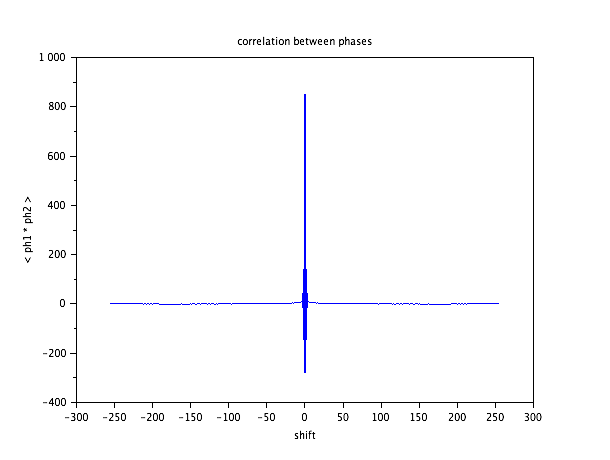Note that the long-range phase correlations are gone, but that there are strong nearby correlations.  This is the effect of using a window, which introduces a strong coupling between nearby frequency components (the price to pay in order to suppress the far away frequency correlation coupling, also known as leakage).  Indeed does the frequency resolution deteriorate under the use of windowing, as we saw.  Deteriorating frequency resolution is nothing else but a strong coupling between nearby frequency components.

We see that effect also in the amplitude behaviour: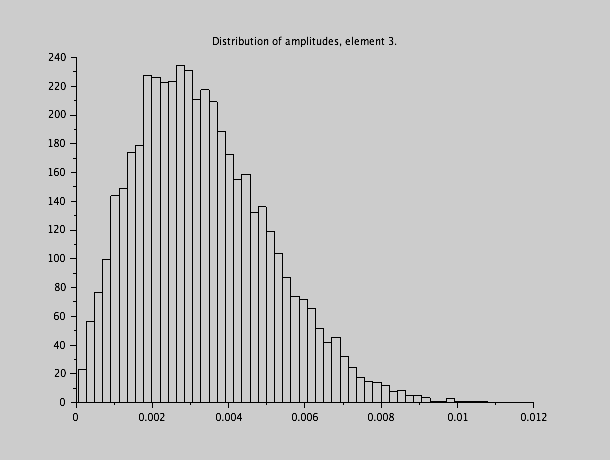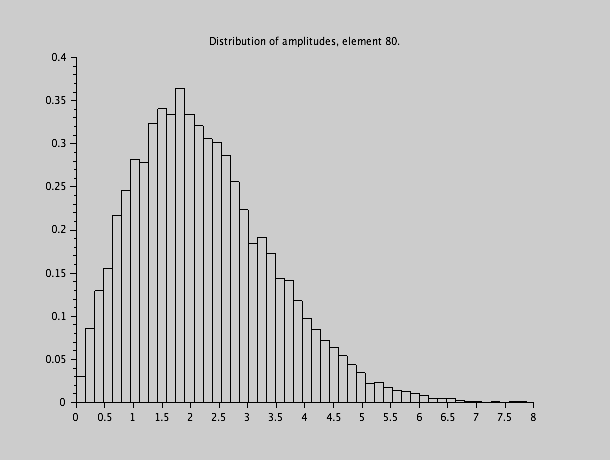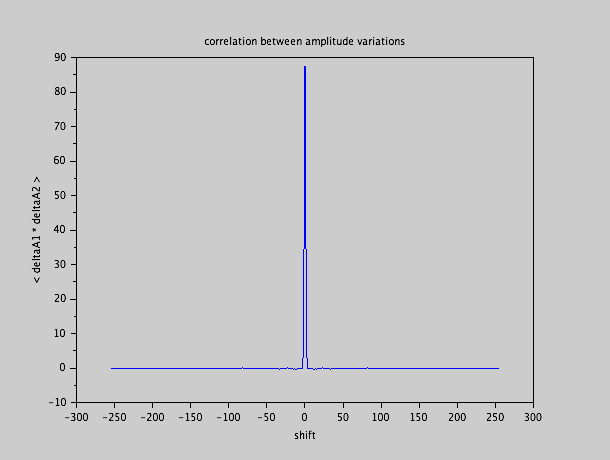As we can clearly see, this correlation function shows a strong correlation of phase deviations within a narrow peak.  But the long range correlations are absent.

Looking at the averages per frequency component, we have finally: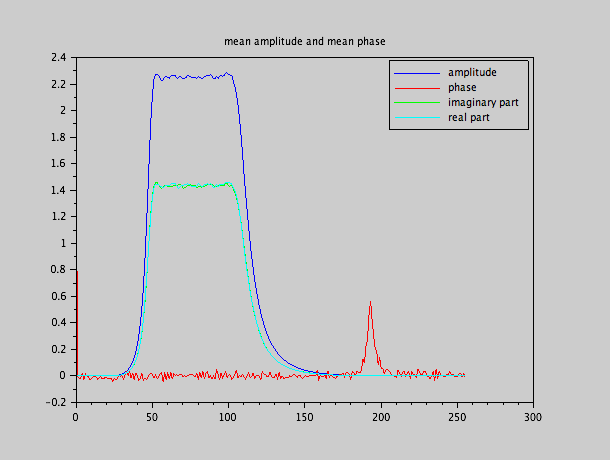As we see, there is no problem with the real part any more.  But not everything is perfect.  The funny little peak in the phase average around 195 is such an example.

Nevertheless, using a Blackman window in this case has allowed us to get at least a much better estimate of the amplitudes (and hence of the spectral power density) than when applying naively the Bartlett periodogram averaging technique.

### Conclusion

The standard method of the averaged periodogram is a useful technique to obtain estimates of the spectral power density of a noise signal, but there are subtleties involved which can lead to wrong results in particular cases.  It takes some expertise to make sure that the spectral density estimator of a noise signal is adequate and doesn't excessively suffer from one or another problem such as leakage.

Apart from the standard periodogram technique that we illustrated here, there are several other spectral estimation techniques that can or cannot be more adequate in particular cases.  Most of the time, these other techniques can only apply if in one way or another some extra knowledge of the noise ensemble.